# Stock Liao information

— Basic knowledge of stocks|Introduction to basics of stocks|Stock learning|Basic knowledge of stocks
Mobile access：m.liaochihuo.com

# The article "Machine Learning" understands the commonly used evaluation indicators of classification algorithms

Release Time:2021-04-28 Topic:Technical indicators with an accuracy of 100 Reading：247 Navigation：Stock Liao information > Mother and child > The article "Machine Learning" understands the commonly used evaluation indicators of classification algorithms

# Foreword

The evaluation index is to input the same data into different algorithm models, or the same algorithm model with different parameters, and give a quantitative index of the quality of this algorithm or parameter.

In the process of model evaluation, it is often necessary to use a variety of different indicators for evaluation. Among the many evaluation indicators, most of the indicators can only one-sidedly reflect part of the performance of the model. If the evaluation indicators cannot be used reasonably, not only cannot Find the problem of the model itself, and will draw wrong conclusions.

Recently, I happened to be doing text classification work, so I went through the evaluation indicators of machine learning classification tasks again. This article will introduce in detail the commonly used evaluation indicators for machine learning classification tasks: Accuracy, Precision, Recall, Precision-Recall Curve, F1 Score, Confuse Matrix, ROC, AUC.

# Accuracy

Accuracy is the most primitive evaluation index in classification problems. The definition of accuracy is

the percentage of the results that are correct in prediction to the total sample, and the formula is as follows: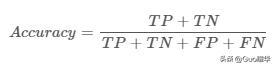Accuracy rate

Where:

-True Positive (

TP): a positive sample predicted by the model to be positive;

-False Positive (

FP): negative samples predicted to be positive by the model;

-False Negative (

FN): positive samples predicted to be negative by the model;

-True Negative (

TN): Negative samples predicted to be negative by the model;

However, the accuracy rate evaluation algorithm has an obvious drawback, that is, when the data types are not balanced, especially when there are extremely biased data, the accuracy rate evaluation index cannot objectively evaluate the pros and cons of the algorithm. . For example, the following example:

In the test set, there are 100 samples, 99 counterexamples, and only 1 positive example. If my model indiscriminately predicts a negative example for any sample, then the accuracy of my model is 0.99, which is very good from a numerical point of view, but in fact, such an algorithm does not have any predictive power, so We should consider whether there is a problem with the evaluation index, and then we need to use other evaluation indexes for comprehensive evaluation.

# Precision, Recall

Precision rate (Precision) is also called

precision rate, it is

in terms of forecast results, and its meaning is

The probability of the sample that is actually positive among all the samples that are predicted to be positive means how certain we can predict the result of the positive sample, the formula is as follows: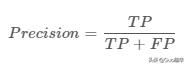Accuracy rate

Precision and accuracy seem to be similar, but two completely different concepts. The accuracy rate represents the accuracy of the prediction in the positive sample results, and the accuracy rate represents the overall prediction accuracy, including both positive samples and negative samples.

Recall rate (Recall) is also called

recall rate, it is

for the original sample, and its meaning is

The probability of being predicted as a positive sample in a sample that is actually positive, the formula is as follows: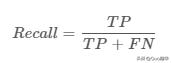Recall rate

Quote the diagram in the Wiki to help explain the relationship between the two.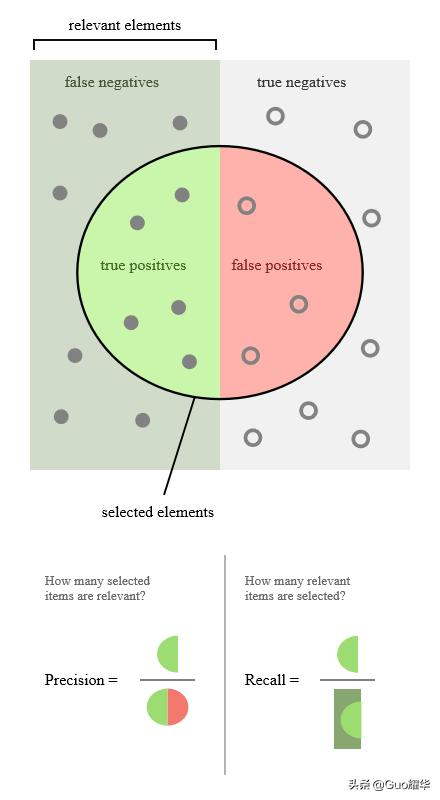Accuracy rate, recall rate

In different application scenarios, our focus is different. For example, when predicting stocks, we are more concerned about the accuracy rate, that is, how much of the stocks we predict to rise, because of those we predicted We invest money in rising stocks. In the scenario of predicting patients, we pay more attention to the recall rate, that is, among those who are really sick, we should predict the wrong situation as few as possible.

Precision and recall are a measure of the ebb and flow of this. For example, in the recommendation system, we want to make the pushed content as interested as possible by all users, so we can only push the content that we hold high, so that some content that users are interested in is omitted, and the recall rate is low; The content that the user is interested in is pushed, so the only way to push all the content is to kill one thousand by mistake instead of letting it go, so the accuracy rate is very low.

In actual engineering, we often need to combine the results of two indicators to find a balance point to maximize comprehensive performance.

# P-R curve

The PR curve (Precision Recall Curve) is exactly the curve describing the changes in accuracy and recall. The PR curve is defined as follows: According to the prediction result of the learner (usually a real value or probability), the test samples are sorted, and the most The samples that may be "positive examples" are ranked first, and the least likely are "positive examples" are ranked behind. In this order, the samples are used as "positive examples" to predict, and the current P value and R value are calculated each time , As shown below: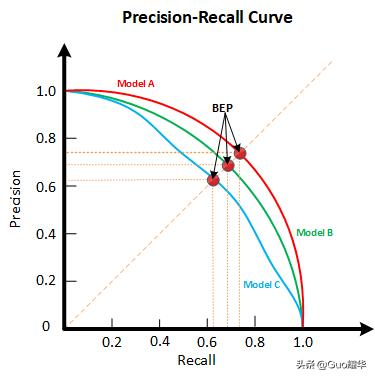P-R curve

How to evaluate the P-R curve? If the P-R curve of one learner A is completely enclosed by the P-R curve of another learner B, it is said that the performance of B is better than that of A. If the curves of A and B cross, whoever has a larger area under the curve will have better performance. But generally speaking, the area under the curve is difficult to estimate, so the "

Balance Point" (Break-Event Point, abbreviated as

BEP) is derived. When P=R, the higher the value of the balance point, the better the performance.

# F1-Score

As mentioned above, the Precision and Recall indicators sometimes trade each other, that is, the higher the accuracy rate, the lower the recall rate. In some scenarios, the accuracy rate and recall rate must be balanced. The most common method is F -Measure, also known as F-Score. F-Measure is the

weighted harmonic average of P and R, namely: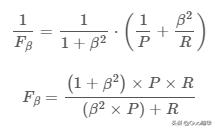F-Score

In particular, when β=1, the common F1-Score is the harmonic average of P and R. When F1 is higher, the performance of the model is better.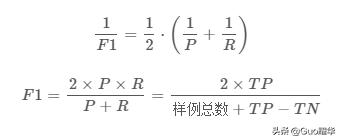F1-Score

# ROC curve

ROC and the AUC mentioned later are very commonly used evaluation indicators in classification tasks. This article will elaborate on them. Some people may have questions. Since there are so many evaluation criteria, why use ROC and AUC?

Because the ROC curve has a very good feature:

When the distribution of positive and negative samples in the test set changes, the ROC curve can remain unchanged. Class Imbalance often occurs in actual data sets, that is, there are many more negative samples than positive samples (or vice versa), and the distribution of positive and negative samples in the test data may also change over time , ROC and AUC can well eliminate the impact of sample category imbalance on the indicator results.

Another reason is that ROC, like the P-R curve mentioned above, is an evaluation index that does not depend on the threshold (Threshold).In a classification model whose output is a probability distribution, if only accuracy, precision, and recall are used as evaluation indicators for model comparison, they must always be based on a given threshold. For different thresholds, each model’s Metrics results will also be different, so it is difficult to get a very confident result.

Before officially introducing ROC, we will introduce two more indicators.

The choice of these two indicators allows ROC to ignore the imbalance of the sample. These two indicators are:

sensitivity (sensitivity) and

specificity (specificity), also called

true rate (TPR) And

False Positive Rate (FPR), the specific formula is as follows.

True Positive Rate (

TPR), also known as sensitivity: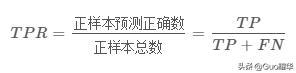In fact, we can find that the sensitivity and recall rate are exactly the same, but the name is changed.

False Negative Rate (

FNR):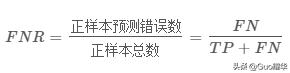False Positive Rate (

FPR):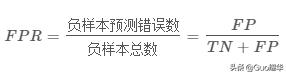True Negative Rate (

TNR), also known as specificity: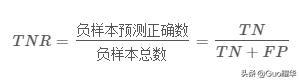Analyzing the above formula in detail, we can see that the sensitivity (true rate) TPR is the recall rate of positive samples, the specificity (true negative rate) TNR is the recall rate of negative samples, and the false negative rate FNR=1-TPR , False positive rate FPR=1-TNR, the above four quantities are all for the prediction results of a single category, so it is not sensitive to whether the overall sample is balanced. For example: Suppose that 90% of the total sample is a positive sample and 10% is a negative sample. In this case, it is unscientific for us to use the accuracy rate for evaluation, but it is possible to use TPR and TNR, because TPR only focuses on how much of the 90% positive sample is predicted to be correct, and 10% negative The sample is irrelevant. Similarly, FPR only focuses on how many of the 10% negative samples are predicted incorrectly, and it has nothing to do with the 90% positive samples. This avoids the problem of sample imbalance.

ROC (Receiver Operating Characteristic) curve, also known as the receiver operating characteristic curve. This curve was first used in the field of radar signal detection to distinguish between signal and noise. Later, people used it to evaluate the predictive ability of the model. The main two indicators in the ROC curve are

True Rate TPR and

False Positive Rate FPR. The benefits of this choice have been explained above. The abscissa is the false positive rate (FPR) and the ordinate is the true rate (TPR). Below is a standard ROC curve.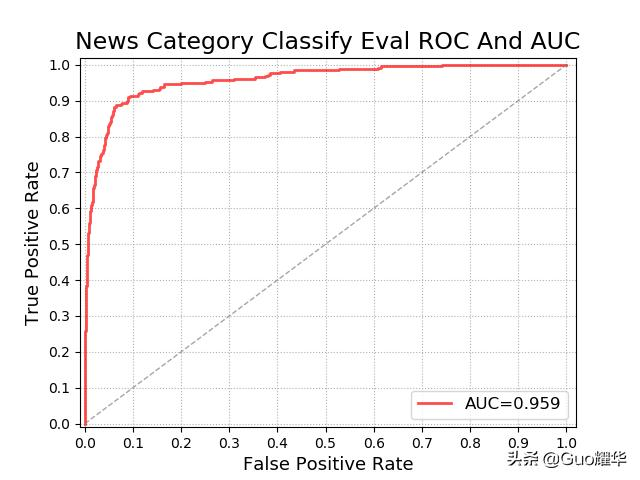ROC curve

Threshold problem

Similar to the previous P-R curve, the ROC curve draws the entire curve by

traversing all thresholds. If we continuously traverse all thresholds, the predicted positive samples and negative samples are constantly changing, and the corresponding ROC curve will also slide along the curve.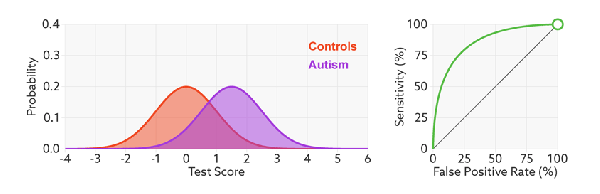We see that changing the threshold only continuously changes the number of predicted positive and negative samples, namely TPR and FPR, but the curve itself has not changed. This makes sense, the threshold does not change the performance of the model.

Judging model performance

So how to judge the ROC curve of a model is good? This still has to return to our purpose: FPR represents the degree to which the model misjudges negative samples, and TPR represents the degree to which the model recalls positive samples. What we hope is of course: the less false positives the negative samples are, the better, and the more positive samples are recalled, the better. So in summary, the higher the

TPR and the lower the FPR (that is, the steeper the ROC curve), the better the performance of the model. Refer to the following dynamic diagram for understanding.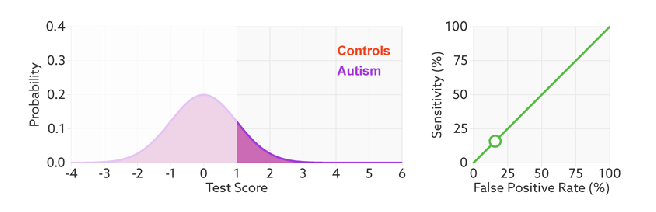That is:

When comparing the performance of models, it is similar to the PR curve. If the ROC curve of a model A is completely enclosed by the ROC curve of another model B, then the performance of B is said to be better than that of A. If the curves of A and B cross, whoever has a larger area under the curve will have better performance.

Ignore sample imbalance

I have explained why the ROC curve can ignore the imbalance of the sample. Let's show how it works again in the form of a dynamic graph. We found that:

No matter how the ratio of red and blue samples is changed, the ROC curve has no effect.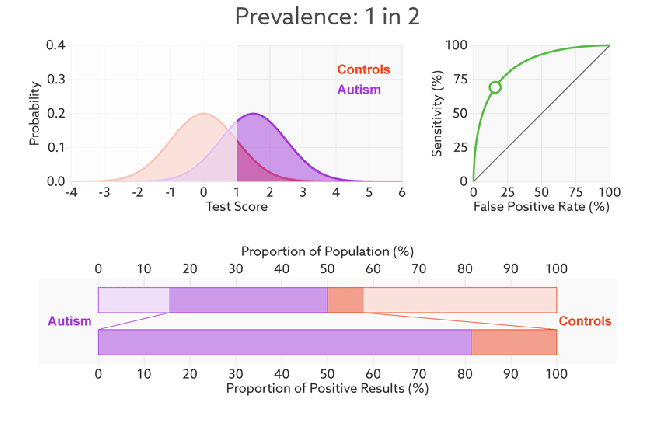# AUC

AUC (Area Under Curve), also known as the area under the curve, is the size of the area under the ROC Curve. As we have mentioned above, the larger the area under the ROC curve, the better the model performance, so AUC is the resulting evaluation index. Generally, the value of AUC is between 0.5 and 1.0, and a larger AUC represents better Performance. If the model is perfect, then its AUC = 1, which proves that all positive examples are ranked in front of the negative examples. If the model is a simple second-class random guessing model, then its AUC = 0.5, if one model is better than the other , The area under the curve is relatively large, and the corresponding AUC value will also be large.

Physical meaning

AUC comprehensively measures the effectiveness of all possible classification thresholds. First of all, the AUC value is a probability value, which can be understood as randomly selecting a positive sample and a negative sample, and the probability that the classifier determines that the score of the positive sample is higher than the score of the negative sample is the AUC value. In short, the larger the AUC value is, the more likely the current classification algorithm is to make the positive sample score higher than the negative sample score, which means it can classify better.

Confusion matrix

The confusion matrix is ​​also called the error matrix, through which the effect of the algorithm can be observed intuitively. Each of its columns is the predicted classification of the sample, and each row is the true classification of the sample (the reverse is also possible). As the name suggests, it reflects the degree of confusion of the classification results. The original of row j of the confusion matrix i is the number of samples that were originally classified into category i but were classified into category j, which can be visualized after calculation: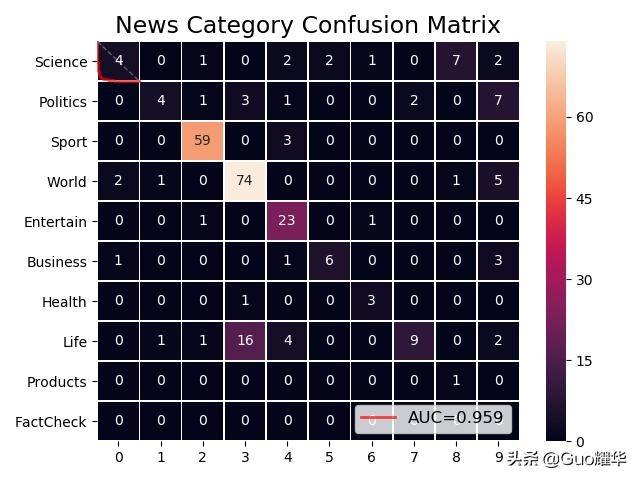# Multi-classification problem

For multi-classification problems, or in two-class classification problems, we sometimes have multiple sets of confusion matrices, such as the results of multiple training or training on multiple data sets, then there are two ways to estimate the global performance , Divided into macro-average and micro-average. Simply understand, macro average is to first calculate the P value and R value of each confusion matrix, and then obtain the average P value macro-P and average R value macro-R, and then calculate Fβ or F1, while micro average is to calculate the confusion matrix The average TP, FP, TN, FN, and then calculate P, R, and then find Fβ or F1. The same is true for other classification indicators, which can be calculated by macro-average/micro-average.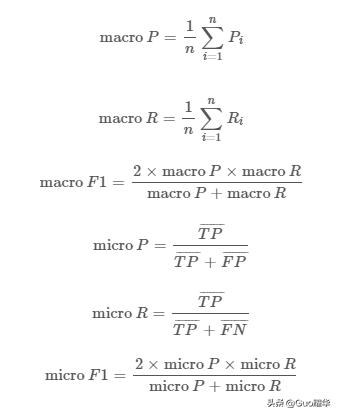It should be noted that in the multi-classification task scenario, if a comprehensive metric must be used,

the macro average is better than the micro average because the macro average is affected by the rare category Bigger. Macro average treats each category equally, so its value is mainly affected by rare categories, while micro average considers every sample in the data set equally, so its value is more affected by common categories.

Author:

Guo Yaohua

Source: http://www.guoyaohua.com

Article Url:https://www.liaochihuo.com/info/535304.html

Label group:[machine learning] [Predictive model] [Recall rate] [roc indicator] [roc] [auc] [threshold

• ### 115 private equity income doubled what did they do right?

Original title 115 private equity companies doubled their earnings, and 13 private equity Shenlinyuan products were listed on the list. What did they...

• ### Private equity positions hit a new high during the year, with more than 80% holding more than 50%

Original title Private equity positions hit a new high during the year, with more than 80% holding more than 50% Source China Securities NetworkWith ...

• ### Buffett's private equity fund positions (Buffett's latest position exposure)

In the first week of November, Ashares rose in shock. Driven by the external market, the major Ashare market indexes turned red every week, and the S...

• ### Ten billion private equity Linyuan responded: Why are you not optimistic about banks and buy bank index?

To invest in stocks, look at the research reports of Golden Kylin analysts, authoritative, professional, timely and comprehensive, to help you tap po...

• ### Big turn over! Linyuan's 20 products earned an average of 19%, and private equity made a huge profit of 1100% this year! _ invest

Its not easy to say Lin Yuan. In the first half of last year, everyone was confused by the sweater war. He made a lot of money from investing in pharm...

• ### Lin Yuan, Dan Bin, Feng Liubaping the hot search list of fund managers are still looking for new investment targets in the pharmaceutical and technology tracks

According to private equity Pai Pai.com data, in the first half of this year, Linyuan Investment Linyuan, Oriental Harbor Dan Bin, Gao Yi Asset Feng L...

• ### Linyuan's three major funds besiege a bull stock, tens of billions of private equity nuggets "just need the mouth" economy

With the start of the Ashare bull market in 2020, Shenzhen Linyuan Investment Management Co., Ltd.The chairman of the company Lin Yuan is very...

• ### The latest ranking of tens of billions of private equity! Perfect circle to win! Linyuan, Dan Bin, Xingshi and other veteran private equity companies are on the list! _ Investment _ Income _

The grand slam of the list refers to the last year, the last three years, and the last five years as of the end of January The companys income is ...